# Geometry - math word problems

1. TriangleTriangle KLM is given by plane coordinates of vertices: K[11, -10] L[10, 12] M[1, 3]. Calculate its area and its interior angles.
2. SimilarityAre two right triangles similar to each other if the first one has a acute angle 70° and second one has acute angle 20°?
3. Reverse Pythagorean theoremGiven are lengths of the sides of the triangles. Decide which one is rectangular: Δ ABC: 77 dm, 85 dm, 36 dm ? Δ DEF: 55 dm, 82 dm, 61 dm ? Δ GHI: 24 mm, 25 mm, 7 mm ? Δ JKL: 32 dm, 51 dm, 82 dm ? Δ MNO: 51 dm, 45 dm, 24 dm
4. VectorCalculate length of the vector v⃗ = (9.75, 6.75, -6.5, -3.75, 2).
5. Segment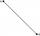Calculate the length of the segment AB, if the coordinates of the end vertices are A[10, -4] and B[5, 5].
6. Circumscribing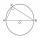Determine the radius of the circumscribed circle to the right triangle with legs 9 cm and 6 cm.
7. SlopeWhat is the slope of the line defined by the equation -2x +3y = -1 ?
8. ClimbOn the road sign, which informs the climb is 8.7%. Car goes 5 km along this road. What is the height difference that car went?
9. OK circleCalculate the radius (circumradius) of the circle described right triangle with hypotenuse long 33 and one cathetus long 17.
10. CenterCalculate the coordinates of the circle center: ?
11. CircleCircle touch two parallel lines p and q; and its center lies on a line a, which is secant of lines p and q. Write the equation of circle and determine the coordinates of the center and radius. p: x-10 = 0 q: -x-19 = 0 a: 9x-4y+5 = 0
12. Perpendicular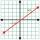What is the slope of the perpendicular bisector of line segment AB if A[9,9] and B[9,-2]?
13. SegmentsWhich of the pairs of numbers on the number line encloses the longest segment: ?
14. Cone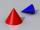If the segment of the line y = -3x +4 that lies in quadrant I is rotated about the y-axis, a cone is formed. What is the volume of the cone?
15. Distance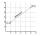Calculate distance between two points X[18; 19] and W[20; 3].
16. CircleWrite the equation of a circle that passes through the point [0,6] and touch the X-axis point [5,0]: ?
17. Perpendicular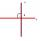Determine the slope of the line perpendicular to the line p: y = -x +4.
18. SlopeWhat is the slope of a line with an inclination 6.06 rad?
19. LineLine p passing through A[-10, 6] and has direction vector v=(3, 2). Is point B[7, 30] on the line p?
20. Center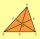In the triangle ABC is point D[1,-2,6], which is the center of the |BC| and point G[8,1,-3], which is the center of gravity of the triangle. Find the coordinates of the vertex A[x,y,z].

Do you have an interesting mathematical word problem that you can't solve it? Submit math problem, and we can try to solve it.

We will send a solution to your e-mail address. Solved examples are also published here. Please enter the e-mail correctly and check whether you don't have a full mailbox.

Please do not submit problems from current active competitions such as Mathematical Olympiad, correspondence seminars etc...